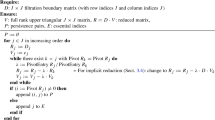# Counting arcs in projective planes via Glynn’s algorithm

## Abstract

An n-arc in a projective plane is a collection of n distinct points in the plane, no three of which lie on a line. Formulas counting the number of n-arcs in any finite projective plane of order q are known for $$n \le 8$$. In 1995, Iampolskaia, Skorobogatov, and Sorokin counted 9-arcs in the projective plane over a finite field of order q and showed that this count is a quasipolynomial function of q. We present a formula for the number of 9-arcs in any projective plane of order q, even those that are non-Desarguesian, deriving Iampolskaia, Skorobogatov, and Sorokin’s formula as a special case. We obtain our formula from a new implementation of an algorithm due to Glynn; we give details of our implementation and discuss its consequences for larger arcs.

This is a preview of subscription content, log in via an institution to check access.

Price excludes VAT (USA)
Tax calculation will be finalised during checkout.

### On 9-regular bipartitions with distinct even parts

V. S. Veena & S. N. Fathima### On the isoperimetric profile of the hypercube

Federico GlaudoUlrich Bauer

## References

1. Batten, L.M.: Combinatorics of Finite Geometries, 2nd edn. Cambridge University Press, Cambridge (1997)

2. Batten, L.M., Beutelspacher, A.: The Theory of Finite Linear Spaces: Combinatorics of Points and Lines. Cambridge University Press, Cambridge (2009)

3. Betten, A., Betten, D.: Linear spaces with at most 12 points. J. Comb. Des. 7, 119–145 (1999)

4. Elkies, N.D.: The moduli spaces of the ten $$10_3$$-configurations. Preprint (2016)

5. Gleason, A.: Finite Fano planes. Am. J. Math. 78(4), 797–807 (1956)

6. Glynn, D.: Rings of geometries. II. J. Combin. Theory Ser. A 49(1), 26–66 (1988)

7. Grünbaum, B.: Configurations of Points and Lines. Graduate Studies in Mathematics, 103. American Mathematical Society, Providence (2009)

8. Hirschfeld, J.W.P., Thas, J.A.: Open problems in finite projective spaces. Finite Fields Appl. 32, 44–81 (2015)

9. Iampolskaia, A., Skorobogatov, A.N., Sorokin, E.: Formula for the number of [9,3] MDS codes. IEEE Trans. Inf. Theory 41(6), 1667–1671 (1995)

10. Kaplan, N., Kimport, S., Lawrence, R., Peilen, L., Weinreich, M.: The number of $$10$$-arcs in the projective plane is not quasipolynomial (in preparation) (2017)

11. Lawrence, R., Weinreich, M.: Counting 10-arcs in the projective plane over a finite field. http://rachellawrence.github.io/TenArcs/

12. McKay, B.D., Piperno, A.: Practical graph isomorphism, II. J. Symb. Comput. 60, 94–112 (2013)

13. Rolland, R., Skorobogatov, A.N.: Dénombrement des configurations dans le plan projectif, In: Proceedings of Arithmetic, Geometry, and Coding Theory (Luminy 1993), 199–207, de Gruyter, Berlin (1996)

14. Sturmfels, B.: Computational algebraic geometry of projective configurations. J. Symb. Comput. 11, 595–618 (1991)

15. Tait, M.: On a problem of Neumann. arXiv:1509.06587 (2015), p. 4.

16. The On-Line Encyclopedia of Integer Sequences, published electronically at https://oeis.org, 2016, Sequence A001200

17. Weibel, C.: Survey of non-Desarguesian planes. Not. Am. Math. Soc. 54(10), 1294–1303 (2007)

## Author information

Authors

### Corresponding author

Correspondence to Nathan Kaplan.

## Rights and permissions

Reprints and Permissions